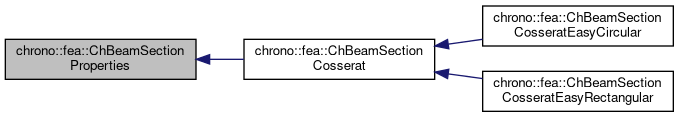chrono::fea::ChBeamSectionProperties Class Referenceabstract

## Description

Base class for properties of all beam sections.

A beam section can be shared between multiple beams. A beam section contains the models for elasticity, plasticity, damping, etc.

#include <ChBeamSection.h>

Inheritance diagram for chrono::fea::ChBeamSectionProperties:[legend]

## Public Member Functions

void SetDrawThickness (double thickness_y, double thickness_z)
Sets the rectangular thickness of the beam on y and z directions, only for drawing/rendering purposes (these thickness values do NOT have any meaning at a physical level, use SetAsRectangularSection()

double GetDrawThicknessY ()

double GetDrawThicknessZ ()

bool IsCircular ()
Tells if the section must be drawn as a circular section instead than default rectangular.

void SetCircular (bool ic)
Set if the section must be drawn as a circular section instead than default rectangular.

Sets the radius of the beam if in 'circular section' draw mode, only for drawing/rendering purposes (this radius value do NOT have any meaning at a physical level, use ChBeamSectionBasic::SetAsCircularSection()

void SetArea (const double ma)
Set the cross sectional area A of the beam (m^2)

double GetArea () const

void SetDensity (double md)
Set the density of the beam (kg/m^3)

double GetDensity () const

virtual void SetAsRectangularSection (double width_y, double width_z)=0
Shortcut: set elastic and plastic constants at once, given the y and z widths of the beam assumed with rectangular shape.

virtual void SetAsCircularSection (double diameter)=0
Shortcut: set elastic and plastic constants at once, given the diameter of the beam assumed with circular shape.

## Public Attributes

double y_drawsize

double z_drawsize

bool is_circular

double Area

double density

The documentation for this class was generated from the following file:
• /builds/uwsbel/chrono/src/chrono/fea/ChBeamSection.h